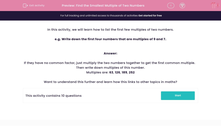# Find the Smallest Multiple of Two Numbers

In this worksheet, students find the first four lowest common multiples of two given numbers.Key stage:  KS 2

Curriculum topic:   Maths and Numerical Reasoning

Curriculum subtopic:   Factors and Multiples

Difficulty level:#### Worksheet Overview

In this activity, we will learn how to list the first few multiples of two numbers.

e.g. Write down the first four numbers that are multiples of 9 and 7.

If they have no common factor, just multiply the two numbers together to get the first common multiple.

Then write down multiples of this number.

Multiples are: 63, 126189252

Want to understand this further and learn how this links to other topics in maths?

### What is EdPlace?

We're your National Curriculum aligned online education content provider helping each child succeed in English, maths and science from year 1 to GCSE. With an EdPlace account you’ll be able to track and measure progress, helping each child achieve their best. We build confidence and attainment by personalising each child’s learning at a level that suits them.

Get started